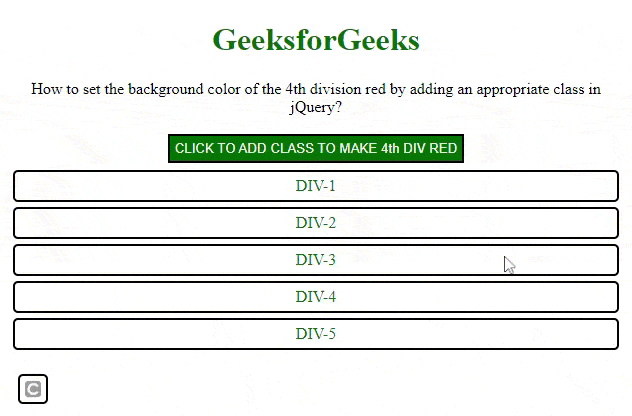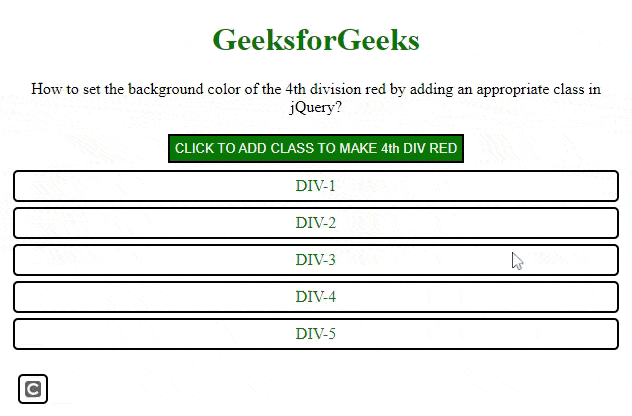Related Articles

# How to set background color of a particular div in jQuery ?

• Last Updated : 19 Jul, 2021

jQuery is one of the powerful libraries of JavaScript that contains many powerful methods for manipulating the DOM or selecting DOM elements and modifying the DOM elements.

In this article, we will set the background color of 4th division to red by adding an appropriate class in jQuery.

Approach: The ith occurrence of an element in the DOM can be located in the DOM using the eq() method. The eq() method returns the ith element of the DOM of that element selected. It considers 0 based indexing.

Syntax:

`\$('selector').eq(index)`

The index is the parameter in the eq() method that can be positive or negative. If it is positive, then it starts from 0. If it is negative, it considers indexing from the end.

Approach 1: Select the body by using jQuery selector. Find the 4th div from the body and then add the class using the addClass() function to the returned jQuery object. Since it is 0 based indexing, we select the div at the 3rd index.

Example:

## HTML

 ```<``html` `lang``=``"en"``>`` ` `<``head``>``    ``<``meta` `charset``=``"UTF-8"``>``    ``<``meta` `http-equiv``=``"X-UA-Compatible"` `            ``content``=``"IE=edge"``>`` ` `    ``<``meta` `name``=``"viewport"` `content``=``        ``"width=device-width, initial-scale=1.0"``>`` ` `    ````    ``<``script` `src``=``"https://code.jquery.com/jquery-3.6.0.min.js"``        ``integrity``=``"sha256-/xUj+3OJU5yExlq6GSYGSHk7tPXikynS7ogEvDej/m4="` `        ``crossorigin``=``"anonymous"``>``    ````     ` `    ``<``style``>``        ``h1 {``            ``color: #006600;``        ``}`` ` `        ``body {``            ``text-align: center;``        ``}`` ` `        ``div {``            ``text-align: center;``            ``padding: 5px;``            ``border: 2px solid black;``            ``border-radius: 5px;``            ``margin: 5px;``            ``color: #006600``        ``}`` ` `        ``#btn {``            ``margin: 2px;``            ``padding: 5px;``            ``border: 2px solid black;``            ``background-color: #006600;``            ``color: whitesmoke;``            ``width: auto``        ``}`` ` `        ``/* The class that turns the div's ``        ``background colour to red */``        ``.newClass {``            ``background-color: red;``            ``color: white;``        ``}``    `````` ` `<``body``>``    ``<``h1``> GeeksforGeeks``    ``<``p``>``        ``How to set the background color ``        ``of the 4th division red by adding ``        ``an appropriate class in jQuery?``    ```` ` `    ``<``button` `id``=``"btn"``>``        ``CLICK TO ADD CLASS TO MAKE 4th DIV RED ``    ````     ` `    ``<``div``> DIV-1 ``    ``<``div``> DIV-2 ``    ``<``div``> DIV-3 ``    ``<``div``> DIV-4 ``    ``<``div``> DIV-5 `` ` `    ``<``script``>``        ``\$(document).ready(function () {``     ` `            ``// Selecting the body using selector``            ``// finding the 4th div i.e 0 based indexing ``            ``// adding a class``            ``\$('#btn').click(function () {``                ``\$('body div').eq(3).addClass('newClass');``            ``})``        ``});``    `````` ` ``

Output:Approach 2: The following example is implemented by selecting the 4th div of the body using the eq() method and styles using css() method.

## HTML

 ```<``html` `lang``=``"en"``>`` ` `<``head``>``    ``<``meta` `charset``=``"UTF-8"``>``    ``<``meta` `http-equiv``=``"X-UA-Compatible"` `        ``content``=``"IE=edge"``>``    ``<``meta` `name``=``"viewport"` `content``=``        ``"width=device-width, initial-scale=1.0"``>`` ` `    ````    ``<``script` `src``=``"https://code.jquery.com/jquery-3.6.0.min.js"``        ``integrity``=``"sha256-/xUj+3OJU5yExlq6GSYGSHk7tPXikynS7ogEvDej/m4="``        ``crossorigin``=``"anonymous"``>``    ````     ` `    ``<``style``>``        ``h1 {``            ``color: #006600;``        ``}`` ` `        ``body {``            ``text-align: center;``        ``}`` ` `        ``div {``            ``text-align: center;``            ``padding: 5px;``            ``border: 2px solid black;``            ``border-radius: 5px;``            ``margin: 5px;``            ``color: #006600``        ``}`` ` `        ``#btn {``            ``margin: 2px;``            ``padding: 5px;``            ``border: 2px solid black;``            ``background-color: #006600;``            ``color: whitesmoke;``            ``width: auto``        ``}``    `````` ` `<``body``>``    ``<``h1``>GeeksforGeeks`` ` `    ``<``p``>``        ``How to set the background color ``        ``of the 4th division red by adding ``        ``an appropriate class in jQuery?``    ```` ` `    ``<``button` `id``=``"btn"``>``        ``CLICK TO ADD CLASS TO MAKE 4th DIV RED``    ```` ` `    ``<``div``> DIV-1 ``    ``<``div``> DIV-2 ``    ``<``div``> DIV-3 ``    ``<``div``> DIV-4 ``    ``<``div``> DIV-5 `` ` `    ``<``script``>``        ``\$(document).ready(function () {`` ` `            ``// Selecting the body using selector``            ``// finding the 4th div i.e 0 based indexing ``            ``// Adding a class``            ``\$('#btn').click(function () {``                ``\$('body div').eq(3).css({ ``                    ``'background-color': 'red', ``                    ``'color': 'white' ``                ``});``            ``})``        ``});``    `````` ` ``

Output:Attention reader! Don’t stop learning now. Get hold of all the important HTML concepts with the Web Design for Beginners | HTML  course.

My Personal Notes arrow_drop_up